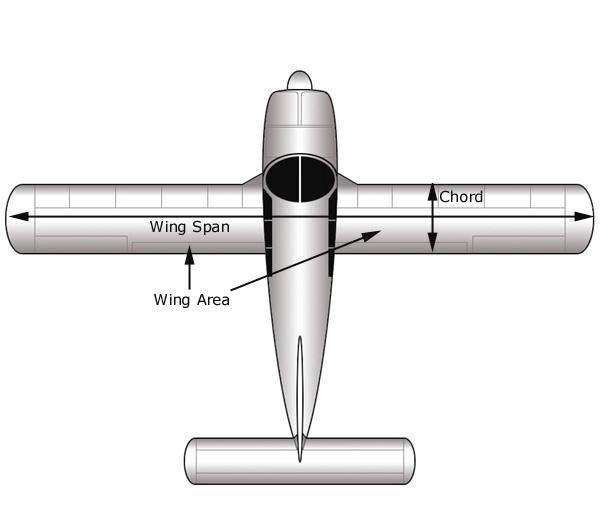In simple terms, Aspect Ratio is the wing span divided by the wing chord. Wing chord is the length of the wing from the wing leading edge to the wing trailing edge. If the wing chord is equal throughout the length of the wing, the formula is: Aspect Ratio = length of the wing/width of the wing     However, because wings come in various shapes, the aspect ratio is the wing span divided by the mean wing chord. If the wing chord is varied throughout the length of the wing, the formula is: Aspect Ratio = wing span˛ / wing area     The aerodynamic aspect ratio is measured between the cores of the wingtip vortices that occur at about 80% of the geometric wingspan when the wing is outside ground effect. The aerodynamic aspect ratio of a wing has a strong inverse effect on lift induced drag.